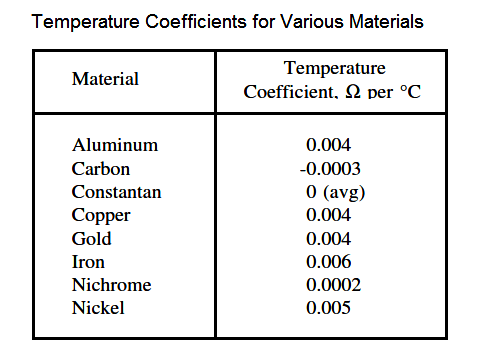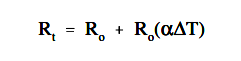# Resistivity

Resistivity is defined as the measure of the resistance a material imposes on current flow. The resistance of a given length of conductor depends upon the resistivity of that material, the length of the conductor, and the cross-sectional area of the conductor, according to Equation.where

R = resistance of conductor, Ω
ρ = specific resistance or resistivity cm-Ω/ft
L = length of conductor, ft
A = cross-sectional area of conductor, cm

The resistivity ρ (rho) allows different materials to be compared for resistance, according to their nature, without regard to length or area. The higher the value of ρ, the higher the resistance.

Table 1 gives resistivity values for metals having the standard wire size of one foot in length and a cross-sectional area of 1 cm.(a) Precise values depend on exact composition of material.

(b) Carbon has 2500-7500 times the resistance of copper.

#### Temperature Coefficient of Resistance

Temperature coefficient of resistance, α (alpha), is defined as the amount of change of the resistance of a material for a given change in temperature. A positive value of α indicates that R increases with temperature; a negative value of α indicates R decreases; and zero α indicates that R is constant. Typical values are listed in Table 2.For a given material, α may vary with temperature; therefore, charts are often used to describe how resistance of a material varies with temperature.

An increase in resistance can be approximated from equation.where

Rt = higher resistance at higher temperatures
R0 = resistance at 0 degc temperature
α = temperature coefficient
∆T = change in temperature

Don't Miss Our Updates
Be the first to get exclusive content straight to your email.
We promise not to spam you. You can unsubscribe at any time.